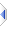# gcd

 Definition: atom a = gcd(object u, atom v=0) Description: Returns the greatest common divisor of two numbers or a sequence of numbers. Comments: The result is always a positive integer (that might still need storing in an atom), except for gcd(0,0) which is 0. atom parameters allow greater precision, but any fractional parts are immediately and deliberately discarded. if the first parameter is a sequence then the second parameter is completely ignored. if the sequence contains only one element, then (floored and unsigned) it is the result, ie gcd({N}) is floor(abs(N)). Ancillary function: integer res = lcm(object m, atom n=0) returns the lowest common multiple, eg lcm(3,4)=lcm(4,6)=12. Implemented in the same file, with similar handling of the parameters to gcd(). Example: ```a = gcd(-9,6) -- a is 3 a = gcd(70000000000000000000, 60000000000000000000000) -- a is 10000000000000000000 a = gcd({57,0,-45,-18,90,447}) -- a is 3 a = gcd({-9.3}) -- a is 9 a = gcd(21,14) -- a is 7 (21=3*7, 14=2*7) a = lcm(21,14) -- a is 42 (=2*21=3*14) ``` Implementation: See builtins\gcd.e (an autoinclude) for details of the actual implementation.